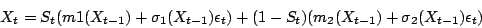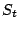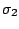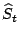# Competing neural networks as models for non stationary financial time series

visiting Charles University of Prague

We consider time series switching between different dynamics or phases, e.g. a generalized mixture of first order nonlinear AR-ARCH models with two dynamicsThe hidden processis a first order Markov chain with values in {0, 1}, the residualsot are i.i.d. with mean 0 and variance 1, the autoregressive,and, and volatility,and, functions are unknown. We first present some conditions that ensure the asymptotic stability( Geometric Ergodicity) of the process and define a version of the likelihood function under mild assumptions. Further, based on the likelihood we investigate the behavior of feedfoward networks for estimating the autoregressive and volatility functions and identifying the changepoints between different phases.

Since the processis not observable, we design a version of the Expectation Maximization algorithm that account for solving the problem numerically. In fact, this algorithm consists of assuming in the Expectation step that the parameters of the networks functions are known and to estimate the. Considering now thethe parameters of the networks functions are derived in theMaximization step. Both steps are iterated until a stopping criterion is satisfied.

Based on these estimations, we construct a trading strategy that we apply on real life data and compare the results with those of the classical Buy and Hold strategy.

2005-05-23